# Introduction

This is a centuries-old game even played by Captain James Cook with his officers on his long voyages. Milton Bradley (now owned by Hasbro) published a version of this game called “Connect Four” in 1974. It is also called “Four-in-a-Row” and “Plot Four.” Two players play this game on an upright board with six rows and seven empty holes. Each player has an equal number of pieces (21) initially to drop one at a time from the top of the board. Then, they will take turns to play and whoever makes a straight line either vertically, horizontally, or diagonally wins.

# Problem Statement

The game is categorized as a zero-sum game. Therefore, the minimax algorithm, which is a decision rule used in AI, can be applied. The project goal is to investigate how a decision tree is applied using the minimax algorithm in this game by Artificial Intelligence.

# · Place in the middle column

If the player can play first, it is better to place it in the middle column. Since the board has seven columns, placing the discs in the middle allows connection to go up vertically, diagonally, and horizontally. In total, there are five possible ways.

# · Make traps for the opponent

One typical way of not losing is to try to block the opponent’s paths toward winning. For example, preventing the opponent from getting a connection of three by placing the disc next to the line in advance to block it. This strategy also prevents the opponent from setting a trap on the player.

# · Make a “7.”

A 7 trap is a name for a strategic move where one positions his disks in a configuration that resembles a 7. With three horizontal disks connected to two diagonal disks branching off from the rightmost horizontal disk. The 7 can be configured in any way, including right way, backward, upside down, or even upside down and backward. This disk formation is a good strategy because it gives players multiple directions to make a connect-four.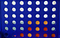The “7” strategy (the yellow-colored discs)

# · Decision Tree

A Decision tree is a tree structure, where each internal node denotes a test on an attribute, each branch represents an outcome of the test, and each leaf node (terminal node) holds a class label. In the example below, one possible flow is as follows: If a person has aged less than 30 and does not eat many pizzas, then that person is categorized as fit. On the contrary, if a person is older than 30, and does not exercise in the morning, then that person is categorized as unfit.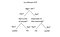A simple decision tree regarding fitness

Decision trees can be applied in different studies, including business strategic plans, mathematics studies, and others. In addition, since the decision tree shows all the possible choices, it can be used in logic games like Connect Four to be served as a look-up table.

# · Decision tree in Connect Four

When the game begins, the first player gets to choose one column among seven to place the colored disc. There are 7 columns in total, so there are 7 branches of a decision tree each time. After the first player makes a move, the second player could choose one column out of seven, continuing from the first player’s choice of the decision tree.

Notice that the decision tree continues with some special cases. First, if both players choose the same column 6 times in total, that column is no longer available for either player. It means that their branches of choice are reduced by one. Second, when both players make all choices (42 in this case) and there are still no 4 discs in a row, the game ends as a draw, and the decision tree stops. Finally, if any player makes 4 in a row, the decision tree stops, and the game ends.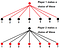Possible moves for each iteration of the Connect Four game shown in the decision tree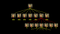Decision tree of Connect Four possible moves

# Minimax algorithm

Minimax algorithm is a recursive algorithm which is used in decision-making and game theory especially in AI game. It provides optimal moves for the player, assuming that the opponent is also playing optimally. For example, considering two opponents: Max and Min playing. Max will try to maximize the value, while Min will choose whatever value is the minimum. The algorithm performs a depth-first search (DFS) which means it will explore the complete game tree as deep as possible, all the way down to the leaf nodes. The algorithm is shown below with an illustrative example.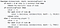minimax algorithm pseudocode

Initially, the algorithm generates the entire game tree and produces the utility values for the terminal states by applying the utility function. For example, in the below tree diagram, let us take A as the tree's initial state. Suppose maximizer takes the first turn, which has a worst-case initial value that equals negative infinity. Then, the minimizer will take the next turn, which has a worst-case initial value that equals positive infinity.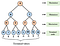Minimax algorithm in tree format — the initial step

First, we consider the Maximizer with initial value = -∞. Each terminal node will be compared with the value of the maximizer and finally store the maximum value in each maximizer node. Take the third row (Maximizer) from the top, for instance.

For node D max(-1, -∞) → max(-1,4) = 4

For Node E max(2, -∞) → max(2, 6) = 6

For Node F max(-3, -∞) → max(-3,-5) = -3

For node G max(0, -∞) → max(0, 7) = 7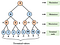Minimax algorithm in tree format — second step

Next, we compare the values from each node with the value of the minimizer, which is +∞.

toFor node B= min(4, 6) = 4

For node C= min(-3, 7) = -3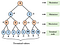Minimax algorithm in tree format — third step

Finally, the maximizer will then again choose the maximum value between node B and node C, which is 4 in this case. Hence, we get the optimal path of play: A → B → D → I.

For node A max(4, -3)= 4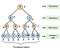Minimax algorithm in tree format — the final step

# AI in Connect Four — Implementing Minimax

Below is a python snippet of Minimax algorithm implementation in Connect Four. In the code, we extend the original Minimax algorithm by adding the Alpha-beta pruning strategy to improve the computational speed and save memory. The figure below is a pseudocode for the alpha-beta minimax algorithm.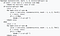minimax algorithm with alpha-beta pruning pseudocode

Note: Https://github.com/KeithGalli/Connect4-Python originally provides the code

I’m just wrapping up and explain the algorithms in Connect Four

Let us take the maximizingPlayer from the code above as an example (From line 136 to line 150). First, the program will look at all valid locations from each column, recursively getting the new score calculated in the look-up table (will be explained later), and finally update the optimal value from the child nodes. Notice that the alpha here in this section is the new_score, and when it is greater than the current value, it will stop performing the recursion and update the new value to save time and memory.

As mentioned above, the look-up table is calculated according to the evaluate_window function below. Here, the window size is set to four since we are looking for connections of four discs. Considering a reward and punishment scheme in this game. If four discs are connected, it is rewarded for a high positive score (100 in this case). When three pieces are connected, it has a score less than the case when four discs are connected. When two pieces are connected, it gets a lower score than the case of three discs connected. Finally, when the opponent has three pieces connected, the player will get a punishment by receiving a negative score. Indicating that it is not an optimal move for the current player.

With the scoring criteria set, the program now needs to calculate all scores for each possible move for each player during the play. The function score_position performs this part from the below code snippet. The AI player will then take advantage of this function to predict an optimal move.

# Conclusions

Looking at how many times AI has beaten human players in this game, I realized that it wins by rationality and loads of information. In this project, the AI player uses a minimax algorithm to check for optimal moves in advance to outperform human players by knowing all possible moves rationally. Interestingly, when tuning the number of depths at the minimax function from high (6 for example) to low (2 for example), the AI player may perform worse. Nevertheless, the strategy and algorithm applied in this project have been proved to be working and performing amazing results.

# References

Galli. (n.d.). KeithGalli/Connect4-Python. GitHub. https://github.com/KeithGalli/Connect4-Python

Nasa, R., Didwania, R., Maji, S., & Kumar, V. (2018). Alpha-beta pruning in mini-max algorithm–an optimized approach for a connect-4 game. Int. Res. J. Eng. Technol, 1637–1641.

## Analytics Vidhya

Analytics Vidhya is a community of Analytics and Data…

Written by

## Jonathan C.T. Kuo

AI | Data Science | DevOps | Classical Music | Projects: (https://github.com/chiatsekuo)

## Analytics Vidhya

Analytics Vidhya is a community of Analytics and Data Science professionals. We are building the next-gen data science ecosystem https://www.analyticsvidhya.com

Written by

## Jonathan C.T. Kuo

AI | Data Science | DevOps | Classical Music | Projects: (https://github.com/chiatsekuo)## Analytics Vidhya

Analytics Vidhya is a community of Analytics and Data Science professionals. We are building the next-gen data science ecosystem https://www.analyticsvidhya.com

## More From Medium

Medium is an open platform where 170 million readers come to find insightful and dynamic thinking. Here, expert and undiscovered voices alike dive into the heart of any topic and bring new ideas to the surface. Learn more

Follow the writers, publications, and topics that matter to you, and you’ll see them on your homepage and in your inbox. Explore

If you have a story to tell, knowledge to share, or a perspective to offer — welcome home. It’s easy and free to post your thinking on any topic. Write on Medium